Open in App
Not now

# Plot Several Curve Segments on the Same Graph

• Last Updated : 30 Jan, 2023

R is a programming language and software environment for statistical computing and graphics. It is widely used among statisticians and data scientists for developing statistical software and data analysis.

R Programming Language is known for its powerful and flexible data visualization capabilities. The base installation of R includes several libraries for creating basic plots and charts, such as the “base” graphics library and the “lattice” library. In addition, there are many other libraries available for R that provide additional visualization capabilities, such as the “ggplot2” library, which is particularly popular for creating complex and highly customizable visualizations.

## R

 `library``(ggplot2)`` ` `# Create sample data``x <- 1:10``y <- x^2`` ` `# Plot data using ggplot2``ggplot``(data = ``data.frame``(x, y)) +``  ``geom_line``(``aes``(x = x, y = y)) +``  ``ggtitle``(``"Curve Plot"``) +``  ``xlab``(``"X values"``) +``  ``ylab``(``"Y values"``)`

Output: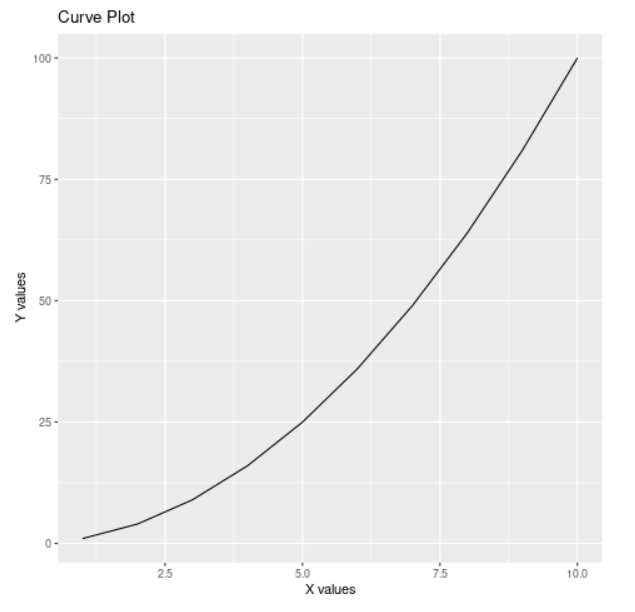Parabola curve which is a single-curve segment

### Curve Segment

A “curve segment” in R generally refers to a portion of a continuous function that is defined over a specific interval of x-values. It is a way of breaking down a curve or function into smaller, manageable parts, and can be useful for understanding how a curve behaves in different regions.

In R, xlim and ylim are used to set the limits of the x and y axis, respectively, when creating a plot. They can be used to zoom in on a specific part of a plot or to remove outliers that might skew the plot. xlim and ylim can be passed to the plot() function or to the coord_cartesian() function in ggplot2 library. The below output shows the formation of the circle using 2 different curves of different colors.

## R

 `# Set up the plot``plot``(0,0, xlim=``c``(-1.5,1.5), ylim=``c``(-1.5,1.5),``     ``xlab=``"x"``, ylab=``"y"``, main=``"Circle"``)`` ` `# Define the radius of the circle``r = 1`` ` `# Define the x and y values ``# for the top half of the circle``x1 = ``seq``(-r,r,length.out=100)``y1 = ``sqrt``(r^2-x1^2)`` ` `# Define the x and y values for``# the bottom half of the circle``x2 = ``seq``(-r,r,length.out=100)``y2 = -``sqrt``(r^2-x2^2)`` ` `# Plot the top half of the circle in red``lines``(x1,y1, col=``"red"``)`` ` `# Plot the bottom half of the circle in blue``lines``(x2,y2, col=``"blue"``)`` ` `# Add a legend to the plot``legend``(``"topright"``, ``c``(``"Top Half"``, ``"Bottom Half"``),``       ``lty=1, col=``c``(``"red"``, ``"blue"``))`

Output: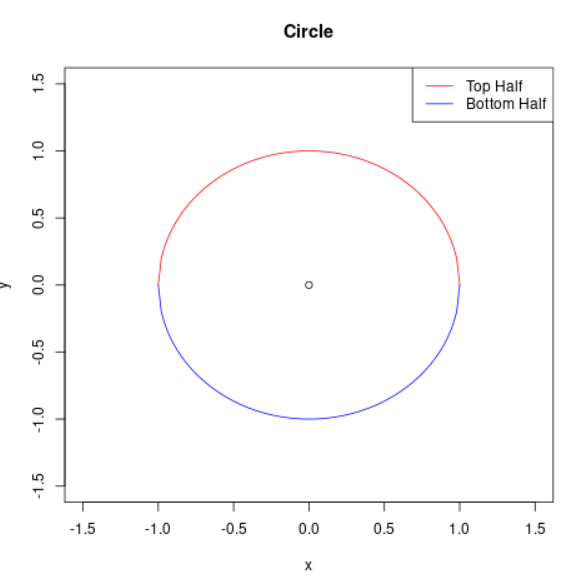Formation of the circle using two different segments

Below is the output generated using the looping statement.

## R

 `# Set up the plot``plot``(1:10, type=``"n"``)`` ` `# Set up the colors``colors <- ``c``(``"red"``, ``"orange"``, ``"yellow"``, ``"green"``,``            ``"blue"``, ``"purple"``, ``"pink"``, ``"brown"``,``            ``"gray"``, ``"black"``)`` ` `# Loop through the colors and plot each curve``for ``(i ``in` `1:10) {``  ``curve``(``sin``(x+i), add=``TRUE``, col=colors[i])``}`

Output: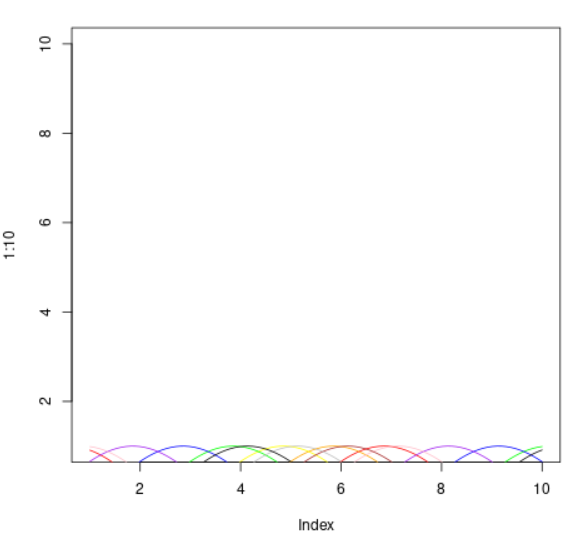Multiple Sine curves on the same graph

Now let’s plot the graph for y = x2 and y = x3 for 1 to 10 and 11 to 20 respectively. In this way, we will be able to plot two curve segments in the same graph.

## R

 `# Create data for curve segments``x1 <- 1:10``y1 <- x1^2``x2 <- 11:20``y2 <- x1^3`` ` `# Plot curve segments on the same graph``plot``(x1, y1, type = ``"l"``, col = ``"blue"``,``     ``lwd = 2, xlim = ``c``(0, 20), ylim = ``c``(0, 1000))``lines``(x2, y2, col = ``"red"``, lwd = 2)`` ` `# Add legend and labels``legend``(``"topright"``, ``c``(``"Curve 1"``, ``"Curve 2"``),``       ``col = ``c``(``"blue"``, ``"red"``), lty = 1, lwd = 2)``xlab``(``"X-axis"``)``ylab``(``"Y-axis"``)`

Output: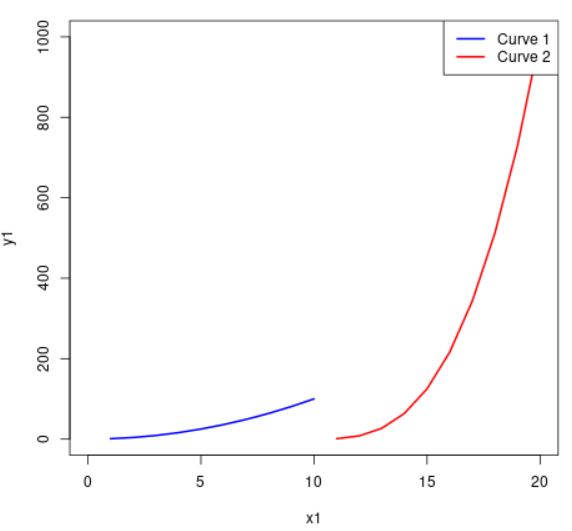Two Curve Segments on the same Graphs

In R, seq() is a function that generates a sequence of numbers. It takes three arguments: the first and last numbers in the sequence, and the step size between numbers.

sin(), cos(), and tan() are trigonometric functions that are commonly used when working with curves. They are used to calculate the sine, cosine, and tangent of an angle, respectively. The angle can be specified in radians or degrees, depending on the context.

For example, you can use the seq() function to create a sequence of x-values and use the trigonometric functions to calculate the corresponding y-values to plot a curve.

## R

 `# Define the range of x values``x <- ``seq``(0, 10, 0.1)`` ` `# Create a vector of y values for each curve``y1 <- ``sin``(x)``y2 <- ``cos``(x)``y3 <- ``tan``(x)``y4 <- ``exp``(x)``y5 <- ``log``(x)``y6 <- ``sin``(x) + ``cos``(x)``y7 <- ``sin``(x) + ``tan``(x)``y8 <- ``cos``(x) + ``tan``(x)``y9 <- ``exp``(x) + ``log``(x)``y10 <- ``sin``(x) + ``cos``(x) + ``tan``(x)`` ` `# Define a vector of colors for each curve``colors <- ``c``(``"red"``, ``"orange"``, ``"yellow"``, ``"green"``,``            ``"blue"``, ``"purple"``, ``"pink"``, ``"brown"``,``            ``"gray"``, ``"black"``)`` ` `# Create a plot with multiple lines``plot``(x, y1, type=``"l"``, col=colors)``lines``(x, y2, col=colors)``lines``(x, y3, col=colors)``lines``(x, y4, col=colors)``lines``(x, y5, col=colors)``lines``(x, y6, col=colors)``lines``(x, y7, col=colors)``lines``(x, y8, col=colors)``lines``(x, y9, col=colors)``lines``(x, y10, col=colors)`` ` `# Add a legend to the plot``legend``(``"topleft"``, ``c``(``"Curve 1"``, ``"Curve 2"``, ``"Curve 3"``,``                    ``"Curve 4"``, ``"Curve 5"``, ``"Curve 6"``,``                    ``"Curve 7"``, ``"Curve 8"``, ``"Curve 9"``,``                    ``"Curve 10"``),``       ``col=colors, lty=1)`

Output: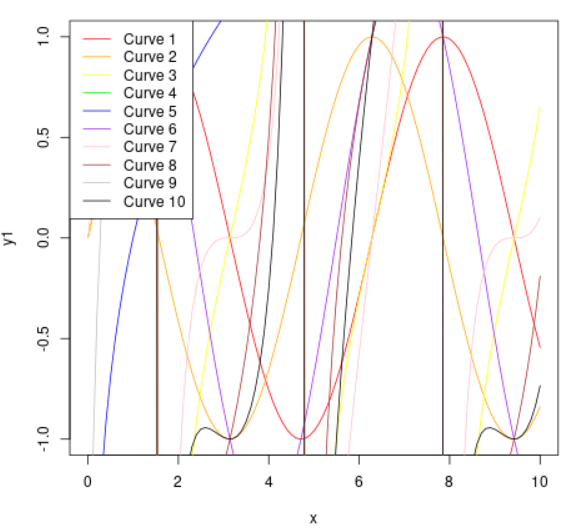Multiple curve segments on the same graph

My Personal Notes arrow_drop_up